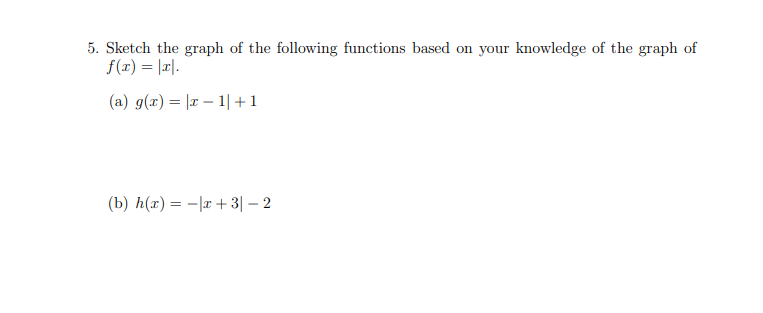# 5. Sketch the graph of the following functions based on your knowledge of the graph off(x) lr(a) g(r) l 1+132(b) h(ar)

Question
1 views

Practice question 5.3help_outlineImage Transcriptionclose5. Sketch the graph of the following functions based on your knowledge of the graph of f(x) lr (a) g(r) l 1+1 32 (b) h(ar) fullscreen
check_circle

Step 1

Graph the following functions based on f(x)=|x|

1. G(x)=|x-1|+1
2. H(x)=|x+3|-2
Step 2

Graph g(x) = |x-1|+1

Graph for g(x) = |x|,

Step 3

Shifting  the graph 1 unit...

### Want to see the full answer?

See Solution

#### Want to see this answer and more?

Solutions are written by subject experts who are available 24/7. Questions are typically answered within 1 hour.*

See Solution
*Response times may vary by subject and question.
Tagged in

### Other